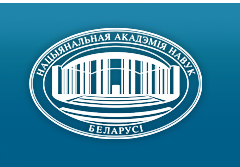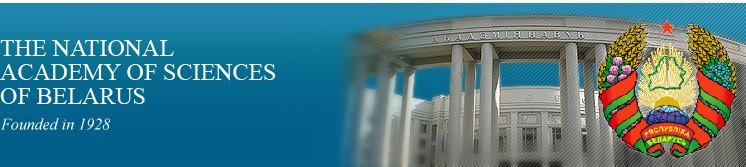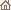Home News About Academy Academy Activities Academy Membership Organizations Publications Conferences Contacts Site Map

# Proc. of the Natl. Academy of Sciences of Belarus, Ser. Phys.-Math. Sci., 1998, No.3/ Publications / Scientific Journals

Proc. of the Natl. Academy of Sciences of Belarus, Ser. Phys.-Math. Sci., 1998, No.3Proceedings of the National Academy of Sciences of Belarus (Vestsi Natsiyanalnai Akademii Navuk Belarusi) SERIES OF PHYSICAL-MATHEMATICAL SCIENCESPublished by The Belaruskaya Navuka Publishing House, Minsk, Republic of Belarus

### Number 3, 1998## CONTENTS

### MATHEMATICS

Grudo Ye. I. †, Demenchuk A. K.
Relations for solving linear algebraic systems in one critical case. pp. 5--6

Summary: Formulas of solutions are given for a systems of linear algebraic equations in one critical case.

Blinova E. I.
A hierarchical algorithm for image denoising. pp. 7--11

Summary: The paper is devoted to recovering of images sampled with random noise. The images are interpreted as functions on the unit square that can take values from the set A = {i/n, 0 £ i £ n}, where n is fixed, only. The hierarchic algorithm consists in detecting parts of the square in which the function is constant, from large parts to small ones. The Ls-risks of recovering are estimated in terms of Besov spaces norms. This estimates can not be improved up to logarithmic factor. The result for d-dimensional case is formulated, too.

Tiounchik A. A.
Minimizing the height of parallel forms of algorithms by the branching method. pp. 12--17

Summary: A method for the construction of the efficient parallel forms of algorithms is proposed. The method generalizes known strategies of partitioning and mapping of the graph models of algorithms onto array processors and provides the minimization of time requirements for the implementation of the algorithms by the processors.

Tuzik A. I.
On the index of a singular integral equation with a finite commutative group of Carleman's direct and reverse shifts. pp. 18--20

Summary: A simple relation for calculating the index of a singular integral equation with a finite commutative group of Carleman's direct and reverse shifts is obtained.

Tishchenko K. I.
On approximation to integer p-adic numbers by algebraic numbers having a bounded degree. pp. 21--24

Summary: The p-adic analog of Wirsing's theorem is obtained.

Ignatenko M. V., Yanovich L. A.
Newton type formulas for the operator interpolation on the spaces of many variable functions. pp. 25--30

Summary: The Newton interpolation formulas for differentiable and nondifferentiable operators defined on the sets of functions of many variables are constructed.

Rovba E. A.
On one orthogonal system of rational functions and the Gauss type quadratures. pp. 31--35

Summary: Quadrature formulas generalizing the classical Gauss formulas of Jakobi nodes with a = -b = 1/2 are given.

Radyno A. Ya.
Degree of convergence of rational approximation depending on the order and type of a function to be approximated. pp. 36--41

Summary: Relation between the rate of rational approximation of an analytic function f and its order and type are established. The corresponding theorems are proved.

Egorov A. D., Zherelo A. V.
Approximate formulas for the class of functional integrals on quasimeasure based on interpolating the measure. pp. 42--45

Summary: Approximate formulas based on interpolation of quasimeasure are constructed for functional integrals w.r.t. quasimeasures.

Vabishchevich P. N., Chuiko M. M.
Difference schemes for one class of systems of convection-diffusion-reaction equations. pp. 46--51

Summary: The boundary value problem for system of two convection-diffusion-reaction equations is considered. For nonstationary differential problem the maximum principle is formulated, a priory estimates are given and a monotonic difference scheme is constructed. It is shown the convergence of difference solution to the exact solution with the first order in the grid norm L1(w).

Shilin A. P.
A singular integral equation with the conjugation in the regular part. pp. 52--54

Summary: A new singular integral equation is solved.

Sobolevski S. L.
On one method of representation of single-valued analytic functions with a discontinuous set of singular points. pp. 55--60

Summary: A single-valued analytic function can be represented in a neighborhood of an isolated singular point with the help of the Lourent series. In particular, if the function has no other singularities, the Lourent series represents it in the whole domain where the function is defined. V. P. Khavin obtained a similar representation by the way of integral series of a special type for single-valued analytic functions with a bounded continuous singular set. In the present paper we obtain necessary and sufficient conditions for the existence of the similar expansion for single-valued analytic functions with a bounded discontinuous singular set.

Tsegelnik V. V.
Selfsimilar solutions of some systems of partial differential equations. pp. 61--63

Summary: Selfsimilar travelling-wave solutions expressed in terms of elementary functions, in terms of elliptic functions or as integrals of the latters for some systems of special nonlinear evolution equations are obtained.

### PHYSICS

Korshunov F. P., Zhdanovich N. E., Marchenko I. G., Troshchinskii V. T.
Investigation of radiation-thermal defects and their influence on the characteristics of silicon diffused p-n-structures. pp. 64--68

Summary: Investigation results about the nature of effective recombination centers induced in diffused p--n-structures on Si after the electron irradiation and subsequent annealing in the temperature range 400--600 °C and their influence on the parameters of these structures in the operation temperature range 77--400 K are presented. It is shown that the recombination in these structures is controlled by the defect with level Ec - 0.32 eV and electron capture cross-section sn > 1· 10-14 cm-2 which contains, seemingly, Al or B.

Hmyl A. A., Anisimovich V. G., Dostanko A. P., Uhov V. A., Boiko L. D.
Physical-mechanical properties of a solid surface treated by electrolyte plasma. pp. 69--74

Summary: Contact resistance, microhardness, brightness and microroughness parameters of steel and brass surfaces were investigated after various polishing techniques. The most smooth surfaces and better physical and mechanical properties are produced by plasmaelectrolytical treatment. Mechanism of improving operation performance is proposed on the base of experimental data obtained with the help of Auger Electron Spectroscopy (AES), X-ray, Scanning Tunnelling Microscope (STM) and Scanning Electron Microscope (SEM).

Vlasov R. A.
Formation of tilted femtosecond solitons in a cubic medium: diffraction-dispersion higher-order effects. pp. 75--77

Summary: The soliton propagation of tilted femtosecond pulses in cubic media is analysed, taking into account the higher-order dispersion-diffraction effects, including the specific "dispersion of diffraction". The latter is shown to exert the crucial influence on the formation of the phase and envelope of a tilted soliton.

Soroko A. V.
Atomic-optic holograms. pp. 78--81

Summary: An analytical solution for off resonant density matrix is presented in such a form, that allows at certain physical conditions to interpret the interaction of ultracold atomic beam with inhomogeneous laser radiation as a passage through a three-dimensional hologram.

Zhur T. A.
Relativistic rotation of a particle in a medium. pp. 82--85

Summary: A system of differential equations, which describes the rotational movement of a particle in post-Newtonian approximation of general relativity, is introduced and integrated. The system was derived on the basis of Papapetrou's equations with some additional conditions on Sab : S0i = K Sij uj. Numeric estimates for some peculiarities of rotational movement of a particle are obtained.

Akimov A. I., Karpei A. L., Savchuk G. K.
Investigation of atomic defects influence on reflection spectra of HTS-phases in thallium based compounds. pp. 86--90

Summary: We report on measurements of far infrared reflection spectra of Tl-based superconducting ceramics with different content of oxygen. Phases 1212, 1223, 1234, 2201, 2212, 2223 are investigated. Phonon modes and their frequencies are determined. It is shown that the phonon near 360 cm-1 is sensitive to the concentration of oxygen in CuO2 plane for the sample with 2201 phase.

Savenko V. S., Ostrikov O. M.
Calculating the strain fields near a twinning duplicate. pp. 91--94

Summary: A method for calculation of the strain fields around two doubles is proposed.

Tishkov V. S., Gaiduk P. I., Shirjaev S.Yu., Larsen A. N.
Structural transformations in alloys Si0.6 Ge0.4 implanted with Ar+ under rapid thermal annealing. pp. 95--99

Summary: Structural transformations produced in strain-relaxed, low-dislocation-density Si0,6 Ge0,4 films by As+ implantation and rapid thermal annealing were studied by transmission electron microscopy and X-ray microanalysis. The type of residual defects was found to be strongly dependent on annealing temperature. Only perfect dislocation loops were observed in implanted layers after annealing at 800 °C. Annealing at higher temperature (900 °C) results in complete removal of irradiation damage accompanied by the formation of GeAs precipitates of monoclinic phase and spherical shape. The results show that the behavior of As in Si-Ge alloys during thermal processing is remarkably different from that in Si. In particular, precipitation of As atoms in Si0,6 Ge0,4 was found at an As concentration of 9 · 1020 cm-3 which is at least one order of magnitude lower than the critical As concentration for As precipitation in Si.

Kazakewich L.A., Lugakov P. F.
Recombination properties of grown-in microdefects in silicon. pp. 100--102

Summary: The temperature and injection dependencies of the charge-carrier lifetime in dislocation-free n-Si were analysed. This allows one to make conclusion about the recombination activity of microdefects of various types. The parameters of the main recombination centres connected with microdefects of A- and B-type were determined.

### INFORMATICS

Kolyada A. A.. Revinskii V. V., Selyaninov M. Yu., Chernyavskii A. F.
Minimal redundant polynomial scalar modular number systems pp. 103--106

Summary: A mathematical formalization of minimum redundant polynomial-scalar modular number systems is given and arithmetics methods for this class of new systems are considered.

Belov D. I., Sadykhov R. Kh.
An algorithm for removing invisible surfaces for the laminated models of three-dimensional objects by approximation with the use of simple polyhedra. pp. 107--110

Summary: An algorithm for removing invisible surfaces for the laminated modes of three-dimensional objects by approximation with the use of simple polyhedra is developed. A new class of simple polyhedra containing convex and star-shaped ones is considered and results of testing and comparative analysis are given.

Lipnitskii S. F., Shibut M. S., Yarmosh N. A.
A hypertext expert training system. I. Data and knowledge model. pp. 111--114

Summary: A conception of expert-tutorial system is proposed that combines quality of a computer adviser, an expert and a handbook. Mathematical models of hypertext database and knowledge base are considered for the control of navigation in hypertext when computer-aided learning is being realized.

Fedyanin D. V., Tikhonenko O. M.
The total demand volume and waiting time distributions for an unreliable queueing system with an infinite number of servers. pp. 115--117

Summary: Summarized demands volume and waiting time characteristics of M/M/¥ queue with simultaneous breakage and renewal of all the servers are determined for the case of service time proportional to the demand length.

Krylenko A. V.
Insensitivity of the stationary distribution of closed queueing networks with rounds of nodes, nonexponential service and several types of customers. pp. 118--122

Summary: Closed networks with N simply nodes with M types of customers and with rounds of nodes are considered. A round of a node depends on the state of the node and the type of a customer. The stationary state distribution is found in multiplicative form and is not dependent on the functional form of service durations of the customers with fixed expected values.

### ABSTRACTS OF DEPOSITED PAPERS

Kiselev A. A.
On generation of subnonattainability. p. 123.

Kiselev A. A., Lebedev A. V.
On information properties of reduced matrices over subnonattainable cardinals. pp. 123--124.

Kiselev A. A.
On nonattainable cardinals. p. 124.

Savenko V. C., Uglov V. V., Ostrikov O. M.
Twinning of bismuth single crystals irradiated with hydrogen ions. p. 124.

Savenko V. C., Ostrikov O. M.
Impurity distribution near twins boundary. p. 125.

In Belarusian Mathematical Society. pp. 126--131.

### SCIENTISTS OF BELARUS

Basov N.G., Burakov V.S., Voitovich A.P., Olekhnovich N.M., Tolkachyov V.A.
Nikolai Aleksandrovich Borisevich (On the occasion of the 75th birthday anniversary). pp. 132--134.
Click
here to read this article in Russian (Windows 1251)

Olekhnovich N.M., Apanasevich P.A., Goncharenko A.M., Petrov N.S., Soika A.K.
Boris Borisovich Boiko (On the occasion of the 75th birthday anniversary). pp. 135--136.
Click here to read this article in Belarusian (Windows 1251)

Apanasevich P.A., Voitovich A.P., Olekhnovich N.M., Kozulin A.V., Korzyuk V.I., Kukharchik P.D.
Aleksandr Fyodorovich Chernyavskii (On the occasion of the 60th birthday anniversary). pp. 137--138.
Click here to read this article in Russian (Windows 1251)/ Publications / Scientific Journals / Back to Top of Page

Designed and maintained by Dr. Nikolai N. Kostyukovich. Last updated: January 30, 1999
Created with assistance of Dr. Ignatii I. Korsak
Copyright © 1998, 1999 The National Academy of Sciences of Belarus
Copyright © 1998 The Belaruskaya Navuka Publishing House